Command Reference : Command Reference

 breakls
Estimation by linear least squares regression with breakpoints.
Syntax
breakls(options) y z1 [z2 z3 ...] [@nv x1 x2 x3 ...]
List the dependent variable first, followed by a list of the independent variables that have coefficients which are allowed to vary across breaks, followed optionally by the keyword @nv and a list of non-varying coefficient variables.
Options
Breakpoint Options

 method=arg (default=“seqplus1”) Breakpoint selection method: “seqplus1” (sequential tests of single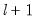versus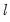breaks), “seqall” (sequential test of all possibleversusbreaks), “glob” (tests of globalvs. no breaks), “globplus1” (tests ofversusglobally determined breaks), “globinfo” (information criteria evaluation), “user” (user-specified break dates). select=arg Sub-method setting (options depend on “method=”).(1) if “method=glob”: Sequential (“seq”) (default), Highest significant (“high”),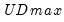(“udmax”),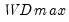(“wdmax”).(2) if “method=globinfo”: Schwarz criterion (“bic” or “sic”) (default), Liu-Wu-Zidek criterion (“lwz”). trim=arg (default=5) Trimming percentage for determining minimum segment size (5, 10, 15, 20, 25). maxbreaks=integer (default=5) Maximum number of breakpoints to allow (not applicable if “method=seqall”). maxlevels=integer (default=5) Maximum number of break levels to consider in sequential testing (applicable when “method=sequall”). breaks="arg" User-specified break dates entered in double quotes. For use when “method=user”. size=arg (default=5) Test sizes for use in sequential determination and final test evaluation (10, 5, 2.5, 1) corresponding to 0.10, 0.05, 0.025, 0.01, respectively heterr Assume regimes specific error distributions in variance computation. commondata Assume a common distribution for the data across segments (only applicable if original equation is estimated with a robust covariance method, “heterr” is not specified).
General Options

 w=arg Weight series or expression. wtype=arg (default=“istdev”) Weight specification type: inverse standard deviation (“istdev”), inverse variance (“ivar”), standard deviation (“stdev”), variance (“var”). wscale=arg Weight scaling: EViews default (“eviews”), average (“avg”), none (“none”).The default setting depends upon the weight type: “eviews” if “wtype=istdev”, “avg” for all others. cov=keyword Covariance type (optional): “white” (White diagonal matrix), “hac” (Newey-West HAC). nodf Do not perform degree of freedom corrections in computing coefficient covariance matrix. The default is to use degree of freedom corrections. covlag=arg (default=1) Whitening lag specification: integer (user-specified lag value), “a” (automatic selection). covinfosel=arg (default=“aic”) Information criterion for automatic selection: “aic” (Akaike), “sic” (Schwarz), “hqc” (Hannan-Quinn) (if “lag=a”). covmaxlag=integer Maximum lag-length for automatic selection (optional) (if “lag=a”). The default is an observation-based maximum of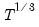. covkern=arg (default=“bart”) Kernel shape: “none” (no kernel), “bart” (Bartlett, default), “bohman” (Bohman), “daniell” (Daniel), “parzen” (Parzen), “parzriesz” (Parzen-Riesz), “parzgeo” (Parzen-Geometric), “parzcauchy” (Parzen-Cauchy), “quadspec” (Quadratic Spectral), “trunc” (Truncated), “thamm” (Tukey-Hamming), “thann” (Tukey-Hanning), “tparz” (Tukey-Parzen). covbw=arg (default=“fixednw”) Kernel Bandwidth: “fixednw” (Newey-West fixed), “andrews” (Andrews automatic), “neweywest” (Newey-West automatic), number (User-specified bandwidth). covnwlag=integer Newey-West lag-selection parameter for use in nonparametric kernel bandwidth selection (if “covbw=neweywest”). covbwoffset=integer (default=0) Apply integer offset to bandwidth chosen by automatic selection method (“bw=andrews” or “bw=neweywest”). covbwint Use integer portion of bandwidth chosen by automatic selection method (“bw=andrews” or “bw=neweywest”). coef=arg Specify the name of the coefficient vector; the default behavior is to use the “C” coefficient vector. prompt Force the dialog to appear from within a program. p Print basic estimation results.
Examples
breakls m1 c unemp
uses the Bai-Perron sequential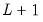versus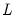tests to determine the optimal breaks in a model regressing M1 on the breaking variables C and UNEMP.
breakls(method=glob, select=high) m1 c unemp
uses the global Bai-Perronversus none tests to determine the breaks. The selected break will be the highest significant number of breaks.
breakls(size=5, trim=10) m1 c unemp
lowers the sequential test size from 0.10 to 0.05, and raises the trimming to 10 percent.
breakls(method=user, break=”1990q1 2010q4”) m1 c @nv unemp
estimates the model with two user-specified break dates. In addition, the variable UNEMP is restricted to have common coefficients across the regimes.
Cross-reference
See “Least Squares with Breakpoints” for discussion. See also “Multiple Breakpoint Tests”.
See Equation::multibreak for estimation of regression equations with breaks.